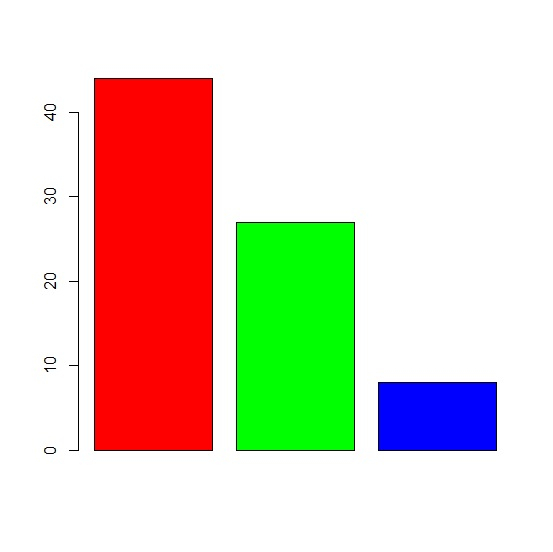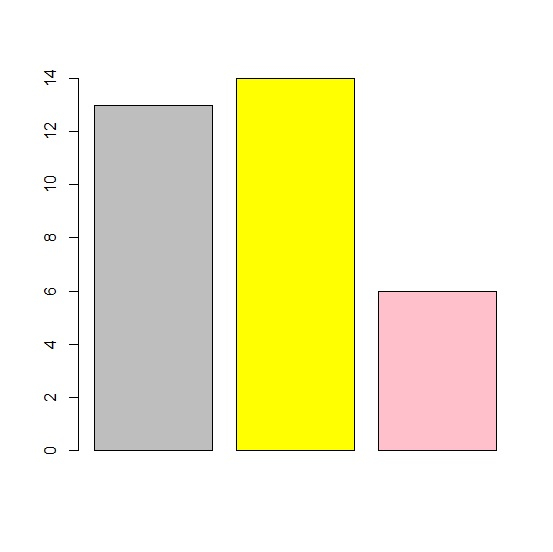# How to manually set the color of each bar in base R bar plot?

To manually set the color of each bar in base R bar plot, we can follow the below steps −

• First of all, create a vector.
• Then create the bar plot with col argument.

## Example 1

Create the vector

Let’s create a vector as shown below −

Live Demo

x<-sample(1:100,3)
x

On executing, the above script generates the below output(this output will vary on your system due to randomization) −

 44 27 8

## Create the bar plot with col argument

Using col argument to set the color of each bar manually −

#### Live Demo

x<-sample(1:100,3)
barplot(x,col=c("red","green","blue"))

#### Output## Example 2

Create the vector

Let’s create a vector as shown below −

Live Demo

y<-rpois(3,10)
y

On executing, the above script generates the below output(this output will vary on your system due to randomization) −

 13 14 6

## Create the bar plot with col argument

Using col argument to set the color of each bar manually −

Live Demo

y<-rpois(3,10)
barplot(y,col=c("grey","yellow","pink"))

#### OutputUpdated on: 14-Aug-2021

552 Views